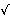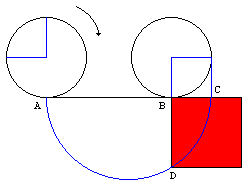# Squaring the Circle

Squaring the Circle, i.e. constructing a square whose area equals that of a given circle, is one of the three famous construction problems of antiquity. Its (negative) solution was eventually obtained in 1882 from the following theorem by the German mathematician Lindemann:

## Theorem

The expression

 (*) A1ea1 + A2ea2 + ... + Anean

in which all coefficients A and a are algebraic, A's are non-zero and a's are all different, cannot vanish.

## Remark

Note that, in the simplest case of a single term ea, the theorem implies that the only point on the graph of y = ex with both x and y rational is (0,1). Although the set of rational points is dense in the plane, the graph of y = ex somehow manages to cut the plane without passing but through just one of them.The theorem is also associated with the French mathematician Hermite(1822-1901) who, in 1873, proved the special case in which the coefficients and exponents were rational numbers. Hermite applied his theorem to prove that e, the base of natural logarithms, is transcendental. (Indeed, if P(e) = 0 for a polynomial with integer coefficients, then we'll get an expression (*) with a's being integers 0, 1, ..., n.)

From the Lindemann's theorem it follows that the number π is also transcendental. This follows from the wonderful Euler's identity

e + 1 = 0

Indeed, on the left we have an expression in the form (*). Since it equals zero, the exponent iπ can't be algebraic. Hence, π is transcendental.

Now returning to the problem of squaring a circle. The area of a circle with radius 1 is exactly π. To construct a square with this area we must be able to construct a segment of lengthπ. Numbers for which it's possible to construct (with a compass and a straightedge) a segment of that length are called constructible. For now, please take my word for it that constructible numbers are algebraic. Also, a product of two constructible numbers is also constructible. Thus ifπ were construcitble so would be π. But then it also would be algebraic in contradiction with the Euler's identity and the Lindemann's theorem.Thus it's impossible to square a circle using a straightedge and a compass; but like the problem of angle trisection, this one can be solved by other means. Have a look at the diagram on the right. Assume a circle of unit radius is rolled half a turn on a straight line. Then the distance between the points A and B will be exactly π. If we draw a semicircle on AC = AB+1 as a diameter, and continue the vertical radius of the right circle to the intersection with the semicircle at a point D, then AB×BC = BD2. Which, of course, solves the famous problem because AB = π and BC = 1.

Note, however, that the distance measurement with the help of a rolling circle is routinely used on modern cars as part of the odometer mechanism.

## References

1. R.Courant and H.Robbins, What is Mathematics?, Oxford University Press, 1996
2. H.Dorrie, 100 Great Problems Of Elementary Mathematics, Dover Publications, NY, 1965.
3. W.Dunham, Journey through Genius, Penguin Books, 1991
4. M.Kac and S.M.Ulam, Mathematics and Logic, Dover Publications, NY, 1968.
5. R.B.Nelsen, Proofs Without Words, MAA, 1993• There are really impossible things...
• An Impossible Frame
• An Impossible Fork
• Structural Constellation
• Squaring a circle
• Trisecting an angle (in general)
• Doubling a cube
• A puzzle of identical twins
• Geometric construction with the straightedge alone
•